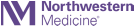# Pediatric Health Library

Health Encyclopedia Explorer
Click a letter to see a list of conditions beginning with that letter.
Click 'Topic Index' to return to the index for the current topic.

# Determining Body Mass Index for Teens

## What is body mass index?

Determining how much your teen should weigh isn't a simple matter of looking at an insurance height-weight chart. It includes considering the amount of bone, muscle, and fat in their body. The amount of fat is the critical measurement.

A good indicator of how much fat your teen carries is the body mass index (BMI). Although it's not a perfect measure, it gives an indirect assessment of how much of your teen's body is composed of fat. BMI is based on weight and height. As a result, it's only a gauge of body fatness. Two people may have the exact same BMI, yet have different amounts of actual body fat.

The formulas below apply to adults only. For children and teens ages 2 to 19 years, the BMI varies by age and sex. An additional step must be done after the BMI has been determined using one of the formulas below. The BMI-for-age percentile is determined by comparing your teen's weight to that of other teens of the same age and sex.

In other words, by plotting your child's BMI value into the  CDC's BMI-for-age growth chart , you can determine if your child is underweight, within normal range, overweight, or obese. Or you can easily calculate it from the CDC's online tool

## To calculate an adult's BMI using the English formula

For example, a person who weighs 165 pounds and is 5 feet 4 inches tall has a BMI of 28. 165 lbs x 703=28(64 inches) x (64 inches).

## To calculate an adult's BMI using the metric formula

A BMI between 25 to 29.9 is considered overweight. Anything over 30 is considered obese. Normal BMI is between 18.5 and 24.9. BMI cut-offs for people of South Asian descent are different.

BMI can be calculated using pounds and inches. BMI= weight in pounds x 703 (height in inches) x (height in inches)

Multiply your weight in pounds by 703.

BMI can be calculated using kilograms and meters. BMI= weight in kilograms (height in meters) x (height in meters)

Divided by your height in meters.